sudheer kumar kotha

•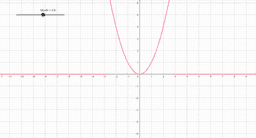Test

Activity

sudheer kumar kotha

•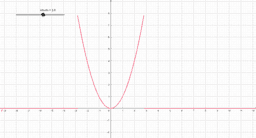Test

Activity

sudheer kumar kotha

•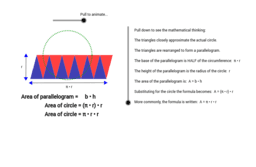Area of a Circle

Activity

sudheer kumar kotha

•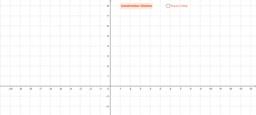Construction

Activity

sudheer kumar kotha

•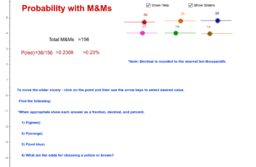Probability with M&Ms

Activity

sudheer kumar kotha

•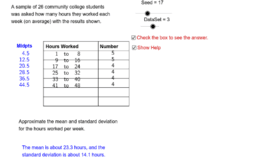Mean and Standard Deviation of Grouped Data

Activity

sudheer kumar kotha

•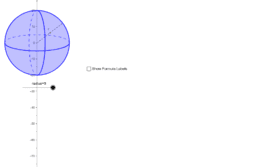Sphere

Activity

sudheer kumar kotha

•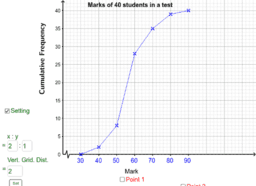Cumulative Frequency Polygon

Activity

sudheer kumar kotha

•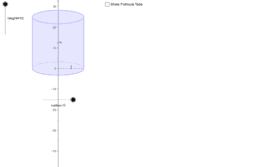Right Circular Cylinder

Activity

sudheer kumar kotha

•Right Circular Cylinder

Activity

sudheer kumar kotha

•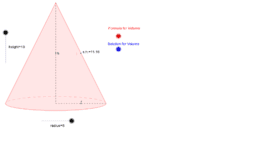Right Circular Cone

Activity

sudheer kumar kotha

•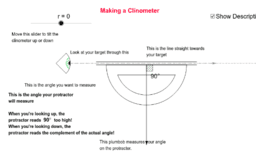Making a Clinometer

Activity

sudheer kumar kotha

•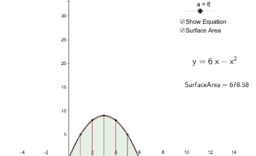Rotating Around the y-axis (Frustums)

Activity

sudheer kumar kotha

•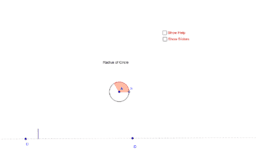Area of Circle = &pi;R<sup>2</sup>.

Activity

sudheer kumar kotha

•Area of Circle: PI * R * R. Why?

Activity

sudheer kumar kotha

•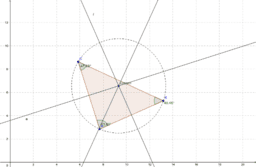CIRCUMCENTER TRIANGLE

Activity

sudheer kumar kotha

•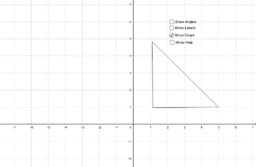Trignonometric Ratio of Some Specific Angle

Activity

sudheer kumar kotha

•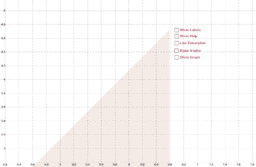right triangles imagine

Activity

sudheer kumar kotha

•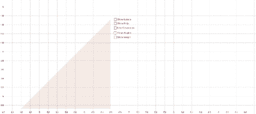right triangles imagine

Activity

sudheer kumar kotha

•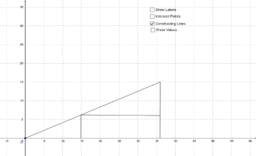Section Formula Activity

Activity

sudheer kumar kotha

•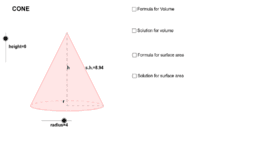Right Circular Cone

Activity

sudheer kumar kotha

•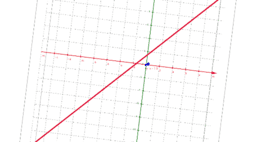Geometrical Meaning of the Zeroes of a Polynomial

Activity

sudheer kumar kotha

•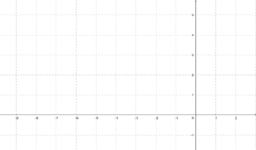Workbench for Trigonometry

Activity

sudheer kumar kotha

•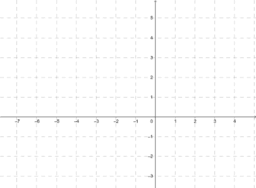Workbench for Arithmetic Progression

Activity

sudheer kumar kotha